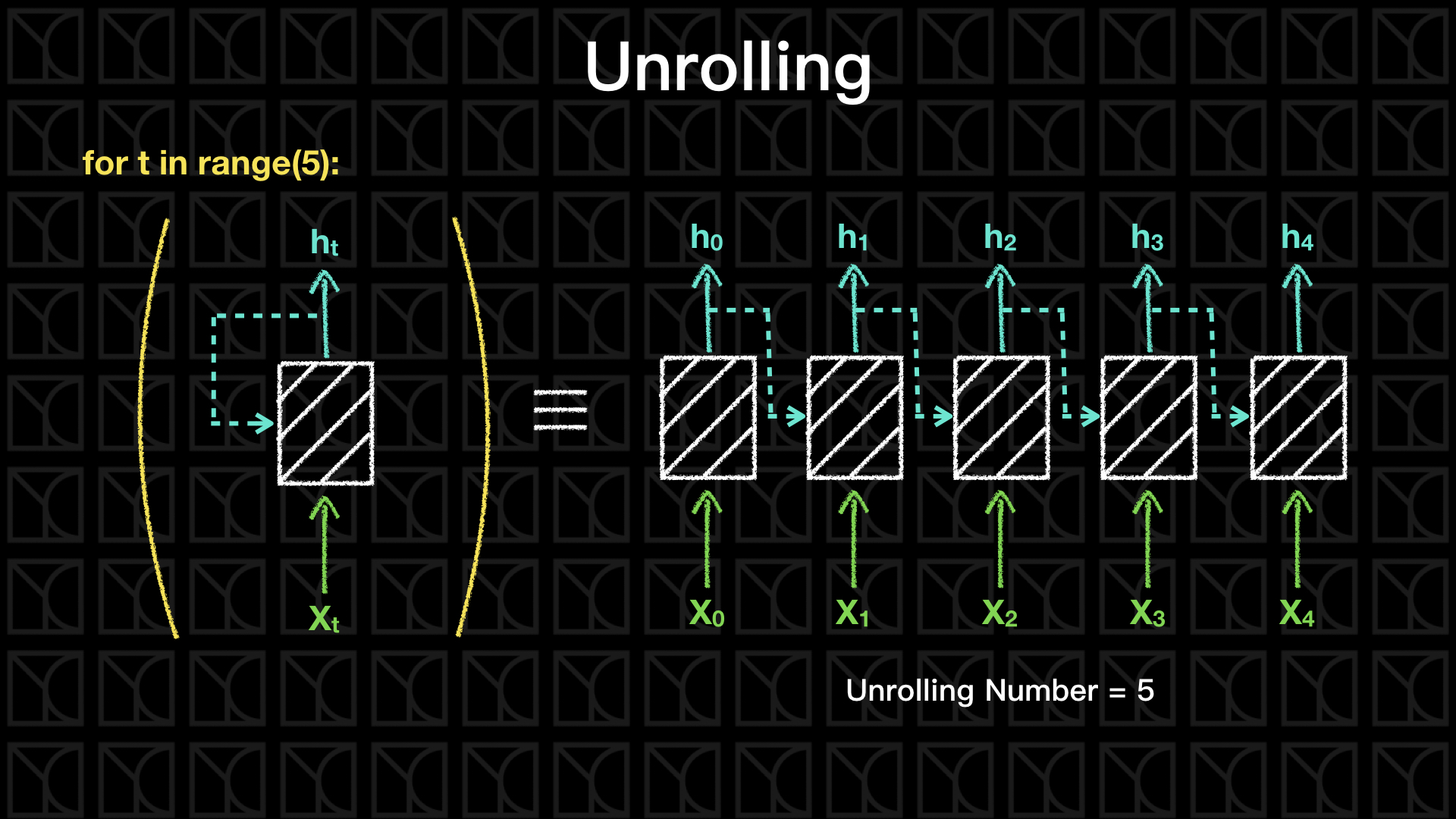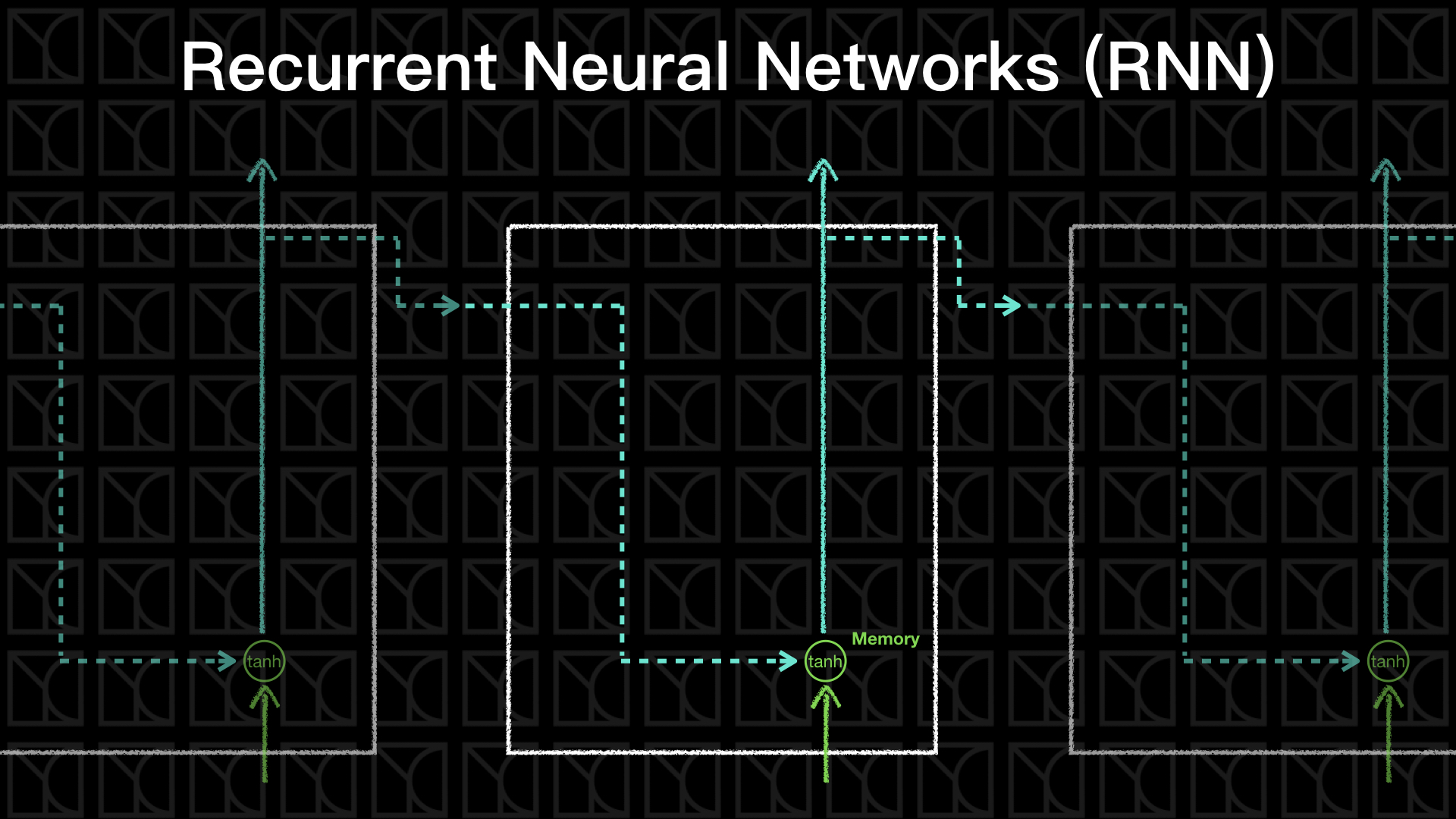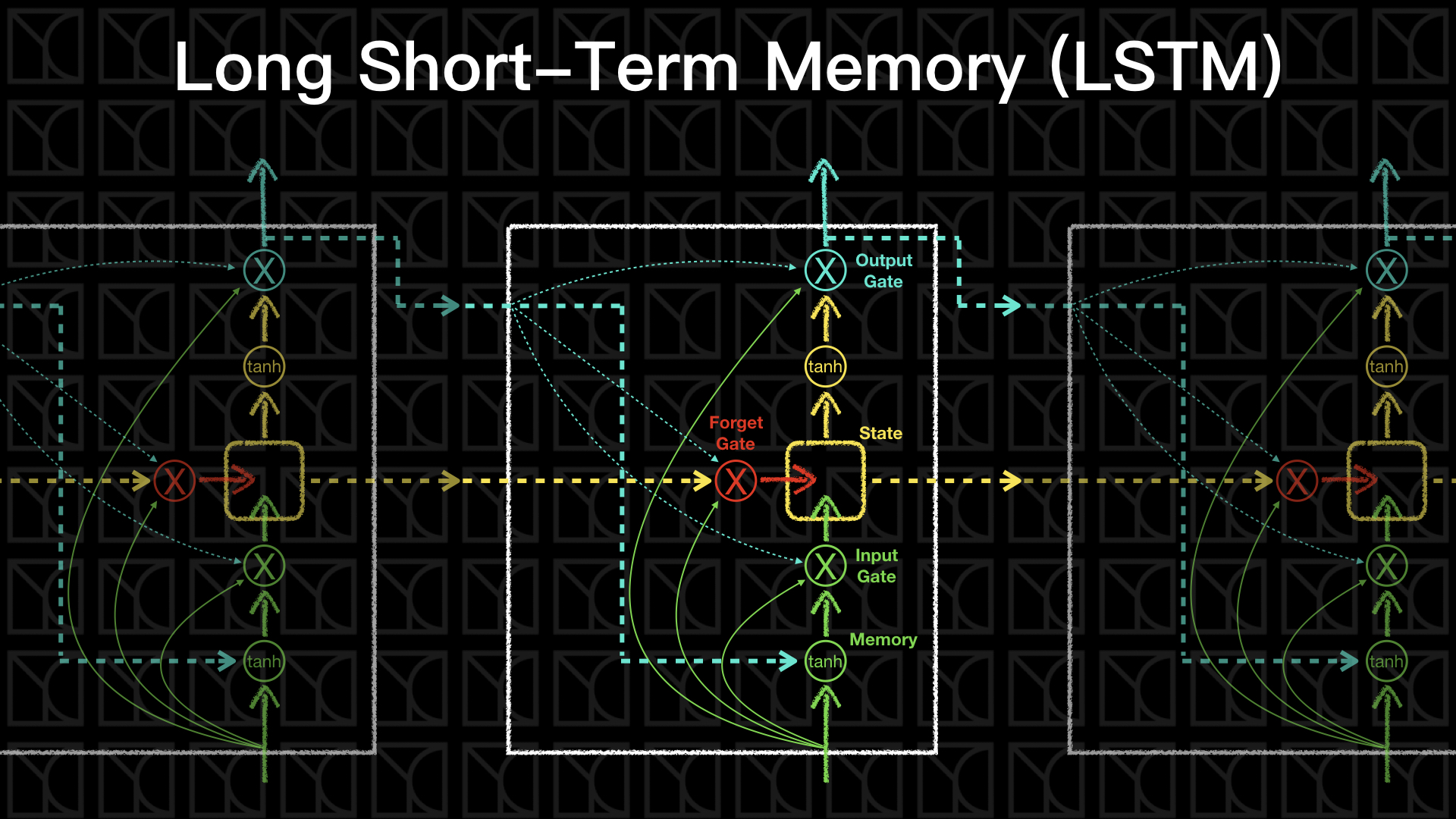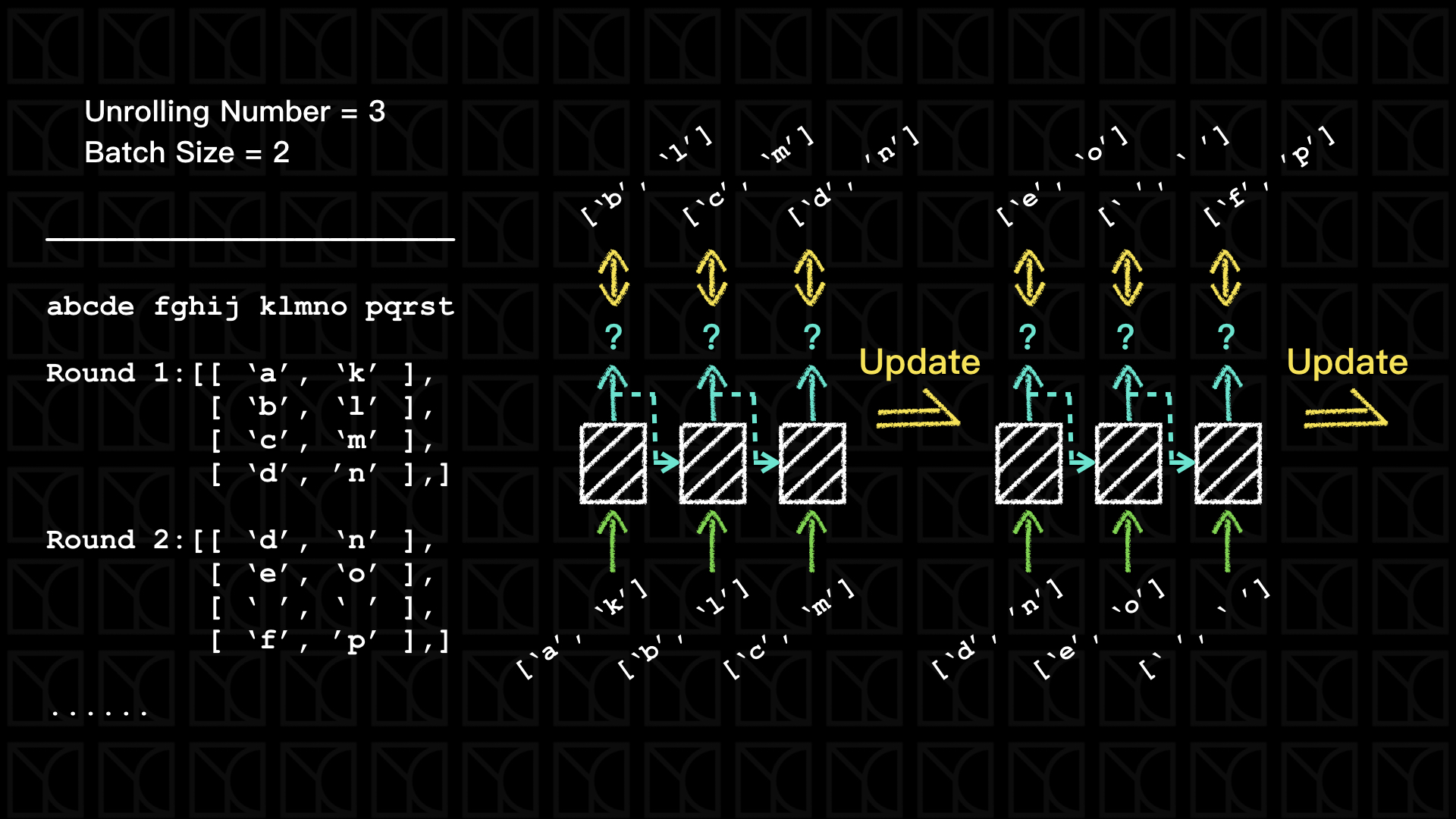# 實作Tensorflow (6)：Recurrent Neural Networks (RNN) and Long Short-Term Memory (LSTM)

Posted on November 25, 2017 in AI.ML. View: 12,443

### 概論RNN$$o_{new}=tanh(i \times W_i + o \times W_o + B)$$

### Long Short-Term Memory (LSTM)

LSTM是現今RNN的主流，它可以解決梯度消失的問題，我們先來看看結構，先預告一下，LSTM是迄今為止這系列課程當中看過最複雜的Neurel Network。$$f_m = tanh (i \times W_{mi} + o \times W_{mo} + B_m)$$

$$f_{state,new} = G_i \times f_m + G_f \times f_{state}$$
$$o_{new} = G_o \times tanh(f_{state,new})$$

$$G_i = Sigmoid (i \times W_{ii} + o \times W_{io} + B_i)$$
$$G_f = Sigmoid (i \times W_{fi} + o \times W_{fo} + B_f)$$
$$G_o = Sigmoid(i \times W_{oi} + o \times W_{oo} + B_o)$$

### 使用LSTM實作文章產生器

  1 2 3 4 5 6 7 8 9 10 11 import os import random import string import zipfile from urllib.request import urlretrieve import time import numpy as np import tensorflow as tf tf.logging.set_verbosity(tf.logging.ERROR) 
  1 2 3 4 5 6 7 8 9 10 11 12 13 14 15 16 17 18 19 20 21 22 23 24 25 26 27 28 29 30 31 32 33 34 35 36 37 38 39 40 41 42 43 44 45 46 47 48 49 50 51 52 53 54 LETTER_SIZE = len(string.ascii_lowercase) + 1 # [a-z] + ' ' FIRST_LETTER_ASCII = ord(string.ascii_lowercase) def maybe_download(url, filename, expected_bytes): """Download a file if not present, and make sure it's the right size.""" if not os.path.exists(filename): filename, _ = urlretrieve(url, filename) statinfo = os.stat(filename) if statinfo.st_size == expected_bytes: print('Found and verified %s' % filename) else: print(statinfo.st_size) raise Exception('Failed to verify ' + filename + '. Can you get to it with a browser?') return filename def read_data(filename): with zipfile.ZipFile(filename) as f: name = f.namelist() data = tf.compat.as_str(f.read(name)) return data def char2id(char): if char in string.ascii_lowercase: return ord(char) - FIRST_LETTER_ASCII + 1 elif char == ' ': return 0 else: print('Unexpected character: %s' % char) return 0 def id2char(dictid): if dictid > 0: return chr(dictid + FIRST_LETTER_ASCII - 1) else: return ' ' print('Downloading text8.zip') filename = maybe_download('http://mattmahoney.net/dc/text8.zip', './text8.zip', 31344016) print('=====') text = read_data(filename) print('Data size %d letters' % len(text)) print('=====') valid_size = 1000 valid_text = text[:valid_size] train_text = text[valid_size:] train_size = len(train_text) print('Train Dataset: size:', train_size, 'letters,\n first 64:', train_text[:64]) print('Validation Dataset: size:', valid_size, 'letters,\n first 64:', valid_text[:64]) 
 1 2 3 4 5 6 7 8 9 Downloading text8.zip Found and verified ./text8.zip ===== Data size 100000000 letters ===== Train Dataset: size: 99999000 letters, first 64: ons anarchists advocate social relations based upon voluntary as Validation Dataset: size: 1000 letters, first 64: anarchism originated as a term of abuse first used against earl1 2 3 4 5 6 7 8 9 10 11 12 13 14 15 16 17 18 19 20 21 22 23 24 25 26 27 28 29 30 31 32 33 34 35 36 37 38 39 40 41 42 43 44 45 46 47 48 49 50 51 52 53 54 55 56 57 58 59 60 61 62 63 64 def characters(probabilities): """Turn a 1-hot encoding or a probability distribution over the possible characters back into its (most likely) character representation.""" return [id2char(c) for c in np.argmax(probabilities, 1)] def batches2string(batches): """Convert a sequence of batches back into their (most likely) string representation.""" s = [''] * batches.shape for b in batches: s = [''.join(x) for x in zip(s, characters(b))] return s def rnn_batch_generator(text, batch_size, num_unrollings): text_size = len(text) ### initialization segment = text_size // batch_size cursors = [offset * segment for offset in range(batch_size)] batches = [] batch_initial = np.zeros(shape=(batch_size, LETTER_SIZE), dtype=np.float) for i in range(batch_size): cursor = cursors[i] id_ = char2id(text[cursor]) batch_initial[i][id_] = 1.0 # move cursor cursors[i] = (cursors[i] + 1) % text_size batches.append(batch_initial) ### generate loop while True: batches = [batches[-1], ] for _ in range(num_unrollings): batch = np.zeros(shape=(batch_size, LETTER_SIZE), dtype=np.float) for i in range(batch_size): cursor = cursors[i] id_ = char2id(text[cursor]) batch[i][id_] = 1.0 # move cursor cursors[i] = (cursors[i] + 1) % text_size batches.append(batch) yield batches # [last batch of previous batches] + [unrollings] # demonstrate generator batch_size = 64 num_unrollings = 10 train_batches = rnn_batch_generator(train_text, batch_size, num_unrollings) valid_batches = rnn_batch_generator(valid_text, 1, 1) print('*** train_batches:') print(batches2string(next(train_batches))) print(batches2string(next(train_batches))) print('*** valid_batches:') print(batches2string(next(valid_batches))) print(batches2string(next(valid_batches))) 
 1 2 3 4 5 6 *** train_batches: ['ons anarchi', 'when milita', 'lleria arch', ' abbeys and', 'married urr', 'hel and ric', 'y and litur', 'ay opened f', 'tion from t', 'migration t', 'new york ot', 'he boeing s', 'e listed wi', 'eber has pr', 'o be made t', 'yer who rec', 'ore signifi', 'a fierce cr', ' two six ei', 'aristotle s', 'ity can be ', ' and intrac', 'tion of the', 'dy to pass ', 'f certain d', 'at it will ', 'e convince ', 'ent told hi', 'ampaign and', 'rver side s', 'ious texts ', 'o capitaliz', 'a duplicate', 'gh ann es d', 'ine january', 'ross zero t', 'cal theorie', 'ast instanc', ' dimensiona', 'most holy m', 't s support', 'u is still ', 'e oscillati', 'o eight sub', 'of italy la', 's the tower', 'klahoma pre', 'erprise lin', 'ws becomes ', 'et in a naz', 'the fabian ', 'etchy to re', ' sharman ne', 'ised empero', 'ting in pol', 'd neo latin', 'th risky ri', 'encyclopedi', 'fense the a', 'duating fro', 'treet grid ', 'ations more', 'appeal of d', 'si have mad'] ['ists advoca', 'ary governm', 'hes nationa', 'd monasteri', 'raca prince', 'chard baer ', 'rgical lang', 'for passeng', 'the nationa', 'took place ', 'ther well k', 'seven six s', 'ith a gloss', 'robably bee', 'to recogniz', 'ceived the ', 'icant than ', 'ritic of th', 'ight in sig', 's uncaused ', ' lost as in', 'cellular ic', 'e size of t', ' him a stic', 'drugs confu', ' take to co', ' the priest', 'im to name ', 'd barred at', 'standard fo', ' such as es', 'ze on the g', 'e of the or', 'd hiver one', 'y eight mar', 'the lead ch', 'es classica', 'ce the non ', 'al analysis', 'mormons bel', 't or at lea', ' disagreed ', 'ing system ', 'btypes base', 'anguages th', 'r commissio', 'ess one nin', 'nux suse li', ' the first ', 'zi concentr', ' society ne', 'elatively s', 'etworks sha', 'or hirohito', 'litical ini', 'n most of t', 'iskerdoo ri', 'ic overview', 'air compone', 'om acnm acc', ' centerline', 'e than any ', 'devotional ', 'de such dev'] *** valid_batches: [' a'] ['an'] 

  1 2 3 4 5 6 7 8 9 10 11 12 13 14 15 16 17 18 19 20 21 22 23 24 def sample_distribution(distribution): """Sample one element from a distribution assumed to be an array of normalized probabilities. """ r = random.uniform(0, 1) s = 0 for i in range(len(distribution)): s += distribution[i] if s >= r: return i return len(distribution) - 1 def sample(prediction): """Turn a (column) prediction into 1-hot encoded samples.""" p = np.zeros(shape=[1, LETTER_SIZE], dtype=np.float) p[0, sample_distribution(prediction)] = 1.0 return p def logprob(predictions, labels): """Log-probability of the true labels in a predicted batch.""" predictions[predictions < 1e-10] = 1e-10 return np.sum(np.multiply(labels, -np.log(predictions))) / labels.shape 

  1 2 3 4 5 6 7 8 9 10 11 12 13 14 15 16 17 18 19 20 21 22 23 24 25 26 27 28 29 30 31 32 33 34 35 36 37 38 39 40 41 42 43 44 45 46 47 48 49 50 51 52 53 54 55 56 57 58 59 60 61 62 63 64 65 66 67 68 69 70 71 72 73 74 75 76 77 78 79 80 81 82 83 84 85 86 87 88 89 90 91 92 93 94 95 96 97 98 99 100 101 102 103 104 105 106 107 108 109 110 111 112 113 114 115 116 117 118 119 120 121 122 123 124 125 126 127 128 129 130 131 132 133 134 135 136 137 138 139 140 141 142 143 144 145 146 147 148 149 150 151 152 153 154 155 156 157 158 159 160 161 162 163 164 165 166 167 168 169 170 171 172 173 174 175 176 177 178 179 180 181 182 183 184 185 186 187 188 189 190 191 192 193 194 195 196 197 198 class LSTM: def __init__(self, n_unrollings, n_memory, n_train_batch, learning_rate=1.0): self.n_unrollings = n_unrollings self.n_memory = n_memory self.weights = None self.biases = None self.saved = None self.graph = tf.Graph() # initialize new grap self.build(learning_rate, n_train_batch) # building graph self.sess = tf.Session(graph=self.graph) # create session by the graph def build(self, learning_rate, n_train_batch): with self.graph.as_default(): ### Input self.train_data = list() for _ in range(self.n_unrollings + 1): self.train_data.append( tf.placeholder(tf.float32, shape=[n_train_batch, LETTER_SIZE])) self.train_inputs = self.train_data[:self.n_unrollings] self.train_labels = self.train_data[1:] # labels are inputs shifted by one time step. ### Optimalization # build neurel network structure and get their loss self.y_, self.loss = self.structure( inputs=self.train_inputs, labels=self.train_labels, n_batch=n_train_batch, ) # define training operation self.optimizer = tf.train.AdagradOptimizer(learning_rate=learning_rate) # gradient clipping # output gradients one by one gradients, v = zip(*self.optimizer.compute_gradients(self.loss)) gradients, _ = tf.clip_by_global_norm(gradients, 1.25) # clip gradient # apply clipped gradients self.train_op = self.optimizer.apply_gradients(zip(gradients, v)) ### Sampling and validation eval: batch 1, no unrolling. self.sample_input = tf.placeholder(tf.float32, shape=[1, LETTER_SIZE]) saved_sample_output = tf.Variable(tf.zeros([1, self.n_memory])) saved_sample_state = tf.Variable(tf.zeros([1, self.n_memory])) self.reset_sample_state = tf.group( # reset sample state operator saved_sample_output.assign(tf.zeros([1, self.n_memory])), saved_sample_state.assign(tf.zeros([1, self.n_memory]))) sample_output, sample_state = self.lstm_cell( self.sample_input, saved_sample_output, saved_sample_state) with tf.control_dependencies([saved_sample_output.assign(sample_output), saved_sample_state.assign(sample_state)]): # use tf.control_dependencies to make sure 'saving' before 'prediction' self.sample_prediction = tf.nn.softmax( tf.nn.xw_plus_b(sample_output, self.weights['classifier'], self.biases['classifier'])) ### Initialization self.init_op = tf.global_variables_initializer() def lstm_cell(self, i, o, state): """"Create a LSTM cell. See e.g.: http://arxiv.org/pdf/1402.1128v1.pdf Note that in this formulation, we omit the various connections between the previous state and the gates.""" ## Build Input Gate ix = self.weights['input_gate_i'] im = self.weights['input_gate_o'] ib = self.biases['input_gate'] input_gate = tf.sigmoid(tf.matmul(i, ix) + tf.matmul(o, im) + ib) ## Build Forget Gate fx = self.weights['forget_gate_i'] fm = self.weights['forget_gate_o'] fb = self.biases['forget_gate'] forget_gate = tf.sigmoid(tf.matmul(i, fx) + tf.matmul(o, fm) + fb) ## Memory cx = self.weights['memory_i'] cm = self.weights['memory_o'] cb = self.biases['memory'] update = tf.matmul(i, cx) + tf.matmul(o, cm) + cb ## Update State state = forget_gate * state + input_gate * tf.tanh(update) ## Build Output Gate ox = self.weights['output_gate_i'] om = self.weights['output_gate_o'] ob = self.biases['output_gate'] output_gate = tf.sigmoid(tf.matmul(i, ox) + tf.matmul(o, om) + ob) ## Ouput output = output_gate * tf.tanh(state) return output, state def structure(self, inputs, labels, n_batch): ### Variable if (not self.weights) or (not self.biases) or (not self.saved): self.weights = { 'input_gate_i': tf.Variable(tf.truncated_normal( [LETTER_SIZE, self.n_memory], -0.1, 0.1)), 'input_gate_o': tf.Variable(tf.truncated_normal( [self.n_memory, self.n_memory], -0.1, 0.1)), 'forget_gate_i': tf.Variable(tf.truncated_normal( [LETTER_SIZE, self.n_memory], -0.1, 0.1)), 'forget_gate_o': tf.Variable(tf.truncated_normal( [self.n_memory, self.n_memory], -0.1, 0.1)), 'output_gate_i': tf.Variable(tf.truncated_normal( [LETTER_SIZE, self.n_memory], -0.1, 0.1)), 'output_gate_o': tf.Variable(tf.truncated_normal( [self.n_memory, self.n_memory], -0.1, 0.1)), 'memory_i': tf.Variable(tf.truncated_normal( [LETTER_SIZE, self.n_memory], -0.1, 0.1)), 'memory_o': tf.Variable(tf.truncated_normal( [self.n_memory, self.n_memory], -0.1, 0.1)), 'classifier': tf.Variable(tf.truncated_normal( [self.n_memory, LETTER_SIZE], -0.1, 0.1)), } self.biases = { 'input_gate': tf.Variable(tf.zeros([1, self.n_memory])), 'forget_gate': tf.Variable(tf.zeros([1, self.n_memory])), 'output_gate': tf.Variable(tf.zeros([1, self.n_memory])), 'memory': tf.Variable(tf.zeros([1, self.n_memory])), 'classifier': tf.Variable(tf.zeros([LETTER_SIZE])), } # Variables saving state across unrollings. saved_output = tf.Variable(tf.zeros([n_batch, self.n_memory]), trainable=False) saved_state = tf.Variable(tf.zeros([n_batch, self.n_memory]), trainable=False) ### Structure # Unrolled LSTM loop. outputs = list() output = saved_output state = saved_state for input_ in inputs: output, state = self.lstm_cell(input_, output, state) outputs.append(output) # State saving across unrollings. with tf.control_dependencies([saved_output.assign(output), saved_state.assign(state)]): # use tf.control_dependencies to make sure 'saving' before 'calculating loss' # Classifier logits = tf.nn.xw_plus_b(tf.concat(outputs, 0), self.weights['classifier'], self.biases['classifier']) y_ = tf.nn.softmax(logits) loss = tf.reduce_mean( tf.nn.softmax_cross_entropy_with_logits( labels=tf.concat(labels, 0), logits=logits)) return y_, loss def initialize(self): self.weights = None self.biases = None self.sess.run(self.init_op) def online_fit(self, X): feed_dict = dict() for i in range(self.n_unrollings + 1): feed_dict[self.train_data[i]] = X[i] _, loss = self.sess.run([self.train_op, self.loss], feed_dict=feed_dict) return loss def perplexity(self, X): sum_logprob = 0 sample_size = len(X)-1 batch_size = X.shape for i in range(batch_size): self.sess.run(self.reset_sample_state) for j in range(sample_size): sample_input = np.reshape(X[j][i], newshape=(1, -1)) sample_label = np.reshape(X[j+1][i], newshape=(1, -1)) predictions = self.sess.run(self.sample_prediction, feed_dict={self.sample_input: sample_input}) sum_logprob += logprob(predictions, sample_label) perplexity = float(np.exp(sum_logprob / batch_size / sample_size)) return perplexity def generate(self, c, len_generate): feed = np.array([[1 if id2char(i) == c else 0 for i in range(LETTER_SIZE)]]) sentence = characters(feed) self.sess.run(self.reset_sample_state) for _ in range(len_generate): prediction = self.sess.run(self.sample_prediction, feed_dict={self.sample_input: feed}) feed = sample(prediction) sentence += characters(feed) return sentence 
  1 2 3 4 5 6 7 8 9 10 11 12 13 14 15 16 17 18 19 20 21 22 23 24 25 26 27 28 29 30 31 32 33 34 35 36 37 38 39 40 41 42 43 44 45 46 47 48 49 50 51 52 53 54 55 # build training batch generator batch_generator = rnn_batch_generator( text=train_text, batch_size=batch_size, num_unrollings=num_unrollings, ) # build validation data valid_batches = rnn_batch_generator( text=valid_text, batch_size=1, num_unrollings=1, ) valid_data = [np.array(next(valid_batches)) for _ in range(valid_size)] # build LSTM model model_LSTM = LSTM( n_unrollings=num_unrollings, n_memory=128, n_train_batch=batch_size, learning_rate=0.9 ) # initial model model_LSTM.initialize() # online training epochs = 30 num_batchs_in_epoch = 5000 valid_freq = 5 for epoch in range(epochs): start_time = time.time() avg_loss = 0 for _ in range(num_batchs_in_epoch): batch = next(batch_generator) loss = model_LSTM.online_fit(X=batch) avg_loss += loss avg_loss = avg_loss / num_batchs_in_epoch train_perplexity = model_LSTM.perplexity(batch) print('Epoch %d/%d: %ds loss = %6.4f, perplexity = %6.4f' % ( epoch+1, epochs, time.time()-start_time, avg_loss, train_perplexity)) if (epoch+1) % valid_freq == 0: print('') print('=============== Validation ===============') print('validation perplexity = %6.4f' % (model_LSTM.perplexity(valid_data))) print('Generate From \'a\': ', model_LSTM.generate(c='a', len_generate=50)) print('Generate From \'h\': ', model_LSTM.generate(c='h', len_generate=50)) print('Generate From \'m\': ', model_LSTM.generate(c='m', len_generate=50)) print('==========================================') print('') 
  1 2 3 4 5 6 7 8 9 10 11 12 13 14 15 16 17 18 19 20 21 22 23 24 25 26 27 28 29 30 31 32 33 34 35 36 37 38 39 40 41 42 43 44 45 46 47 48 49 50 51 52 53 54 55 56 57 58 59 60 61 62 63 64 65 66 67 68 69 70 71 72 73 74 75 76 77  Epoch 1/30: 66s loss = 1.8249, perplexity = 5.6840 Epoch 2/30: 64s loss = 1.5348, perplexity = 5.7269 Epoch 3/30: 63s loss = 1.4754, perplexity = 5.7866 Epoch 4/30: 62s loss = 1.4412, perplexity = 5.3462 Epoch 5/30: 62s loss = 1.4246, perplexity = 5.8845 =============== Validation =============== validation perplexity = 3.7260 Generate From 'a': ah plays agrestiom scattery at an experiments the a Generate From 'h': ht number om one nine six three kg aid rosta franci Generate From 'm': m within v like opens and solepolity ledania as was ========================================== Epoch 6/30: 64s loss = 1.4094, perplexity = 6.0429 Epoch 7/30: 64s loss = 1.3954, perplexity = 5.6133 Epoch 8/30: 63s loss = 1.3905, perplexity = 5.4791 Epoch 9/30: 62s loss = 1.3675, perplexity = 5.7168 Epoch 10/30: 62s loss = 1.3861, perplexity = 5.3937 =============== Validation =============== validation perplexity = 3.5992 Generate From 'a': ands their hypenman sam diversion passes to rouke t Generate From 'h': hash pryess the setuluply see include the grophistr Generate From 'm': merhouses tourism in vertic or influence carbon min ========================================== Epoch 11/30: 64s loss = 1.3782, perplexity = 5.5835 Epoch 12/30: 62s loss = 1.3802, perplexity = 6.0567 Epoch 13/30: 62s loss = 1.3723, perplexity = 6.0672 Epoch 14/30: 62s loss = 1.3729, perplexity = 6.4365 Epoch 15/30: 62s loss = 1.3682, perplexity = 6.2878 =============== Validation =============== validation perplexity = 3.7153 Generate From 'a': ate at decade a july uses mobe on the john press to Generate From 'h': htell yullandi is u one five it naval railandly eng Generate From 'm': ment theory president and much three sinit in harde ========================================== Epoch 16/30: 65s loss = 1.3647, perplexity = 5.5579 Epoch 17/30: 63s loss = 1.3691, perplexity = 5.3885 Epoch 18/30: 64s loss = 1.3535, perplexity = 6.4797 Epoch 19/30: 63s loss = 1.3637, perplexity = 5.8126 Epoch 20/30: 62s loss = 1.3567, perplexity = 5.9839 =============== Validation =============== validation perplexity = 3.6210 Generate From 'a': ate treaty jack a golderazogon develoged civilized Generate From 'h': hyene is ricpstowed dark preferent crurts annivaril Generate From 'm': mer centine all level end of a character of tracks ========================================== Epoch 21/30: 65s loss = 1.3584, perplexity = 6.0557 Epoch 22/30: 63s loss = 1.3535, perplexity = 7.0777 Epoch 23/30: 63s loss = 1.3700, perplexity = 5.7674 Epoch 24/30: 63s loss = 1.3609, perplexity = 6.1226 Epoch 25/30: 64s loss = 1.3663, perplexity = 6.2711 =============== Validation =============== validation perplexity = 3.6048 Generate From 'a': an vary palest in some live halleten converting to Generate From 'h': heper could use that the l bidging the five zero th Generate From 'm': mer yort can the real forexanded or rather then for ========================================== Epoch 26/30: 66s loss = 1.3551, perplexity = 6.1640 Epoch 27/30: 65s loss = 1.3586, perplexity = 6.3620 Epoch 28/30: 65s loss = 1.3744, perplexity = 5.5748 Epoch 29/30: 64s loss = 1.3634, perplexity = 6.0498 Epoch 30/30: 63s loss = 1.3671, perplexity = 6.2313 =============== Validation =============== validation perplexity = 3.4751 Generate From 'a': an one brivistrial empir thorodox to an of one city Generate From 'h': ho wing two he wonders marding where never boat lit Generate From 'm': mptemeignt linerical premore logical boldving on ch ========================================== 

 1 print(model_LSTM.generate(c='t', len_generate=1000)) 
 1 th the oppose asia college on all of indirect i suicide upse angence and including khazool cashle with jeremp of the case hasway was catiline tribui s law can be wounds to free from an eventually locations university colid for admirum syn semition goths display the might the official up it alder stowinity name like or day elenth names and lesk external links a loons for have the genione e elevang cress leven isbn effects on cultural leave to oldincil he hokerzon blacklomen with the known resolvement of literated by college founded to families in ak urke player jain of highling fake state a first o al reason into the son then mmpt one nine three three npunt university unexal and currently amnyanipation behavion from ber and ii variety of the gupife number topan has one three zero z capital prime genary brown one nine five nine so universities country recipient the vegetables bether form the distinct de plus out as a first a johnson quicky s remain which an death to anti in panibus series